Practice Test: Civil Engineering (CE)- 10

# Practice Test: Civil Engineering (CE)- 10 - Civil Engineering (CE)

Test Description

## 65 Questions MCQ Test GATE Civil Engineering (CE) 2023 Mock Test Series - Practice Test: Civil Engineering (CE)- 10

Practice Test: Civil Engineering (CE)- 10 for Civil Engineering (CE) 2023 is part of GATE Civil Engineering (CE) 2023 Mock Test Series preparation. The Practice Test: Civil Engineering (CE)- 10 questions and answers have been prepared according to the Civil Engineering (CE) exam syllabus.The Practice Test: Civil Engineering (CE)- 10 MCQs are made for Civil Engineering (CE) 2023 Exam. Find important definitions, questions, notes, meanings, examples, exercises, MCQs and online tests for Practice Test: Civil Engineering (CE)- 10 below.
Solutions of Practice Test: Civil Engineering (CE)- 10 questions in English are available as part of our GATE Civil Engineering (CE) 2023 Mock Test Series for Civil Engineering (CE) & Practice Test: Civil Engineering (CE)- 10 solutions in Hindi for GATE Civil Engineering (CE) 2023 Mock Test Series course. Download more important topics, notes, lectures and mock test series for Civil Engineering (CE) Exam by signing up for free. Attempt Practice Test: Civil Engineering (CE)- 10 | 65 questions in 180 minutes | Mock test for Civil Engineering (CE) preparation | Free important questions MCQ to study GATE Civil Engineering (CE) 2023 Mock Test Series for Civil Engineering (CE) Exam | Download free PDF with solutions
 1 Crore+ students have signed up on EduRev. Have you?
Practice Test: Civil Engineering (CE)- 10 - Question 1

### “Wanted a two bedroom flat in the court area for immediate possession” - An Advertisement. Assumptions: I. Flats are available in the court area II. Some people will respond to the advertisement III. It is a practice to give such an advertisement

Detailed Solution for Practice Test: Civil Engineering (CE)- 10 - Question 1

The person needs a flat in court area. It may be or may not be possible to have a court area.
The person hopes that he will get the room because if available people will respond to the advertisement.
It may or may not be practice to give such an advertisement and nothing can be said because of insufficient information in the question.

Practice Test: Civil Engineering (CE)- 10 - Question 2

### Which of the following is most similar in meaning to the given wordDIRE

Practice Test: Civil Engineering (CE)- 10 - Question 3

### In the question given below, the sentence has a part printed in bold. That part may contain a grammatical error. Replace that part with four choices given below:The famous playwright has been in the sick bed from the last one week

Practice Test: Civil Engineering (CE)- 10 - Question 4
He always _________ you in his letters
Practice Test: Civil Engineering (CE)- 10 - Question 5
His health ____________ under the pressure of work
Practice Test: Civil Engineering (CE)- 10 - Question 6
A, B and C shoots to hit a target. If A hits the target 4 times in 5 trials, B hits in 3 times in 4 trials and C hits it 2 times in 3 trials. What is the probability that the target is hit by at least 2 persons?
Detailed Solution for Practice Test: Civil Engineering (CE)- 10 - Question 6
Probability that A hits the target = 4/5
Probability that B hits the target = 3/4
Probability that C hits the target = 2/3
P (at least 2) = P(2) + P(3)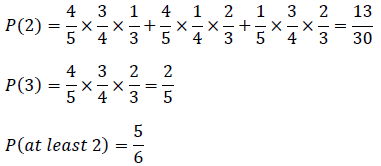Practice Test: Civil Engineering (CE)- 10 - Question 7
Two liquids are mixed in the ratio 4:3 and the mixture is sold at Rs 20 with a profit of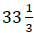% If the first liquid is costlier than the second by Rs 7. Find the sum of costs of both the liquids.
Detailed Solution for Practice Test: Civil Engineering (CE)- 10 - Question 7
Profit =%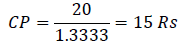Cost of 1 unit of liquid 1 = x+7
Cost of 1 unit of liquid 2 = x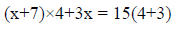x = 11
x+7 = 18
Total value of the prices = 11+18 = 29
Practice Test: Civil Engineering (CE)- 10 - Question 8
Henry and Ford worked together to complete a task in 10 days. Had Henry worked with half of his efficiency and Ford at 5 times of his efficiency it would have taken them to finish the job in 50% of the scheduled time. In how many days can Ford complete the job working alone?
Detailed Solution for Practice Test: Civil Engineering (CE)- 10 - Question 8
Let henry’s one day work be x
Let ford’s one day work be y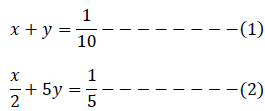Solving 2 equations we get, y = 1/30
Hence, Ford can complete the job working alone in 30 days
Practice Test: Civil Engineering (CE)- 10 - Question 9
A new flag is to be designed with six vertical stripes using some or all of the colours- yellow, green, blue and red. Then the number of ways this can be done such that no two adjacent stripes have the same colour out is:
Detailed Solution for Practice Test: Civil Engineering (CE)- 10 - Question 9
There are 6 stripes. The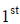stripe can be coloured in 4ways,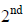in 3,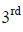in 3 and so on.Thus, total number of ways =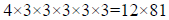Practice Test: Civil Engineering (CE)- 10 - Question 10
Find the value of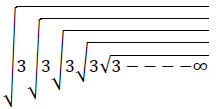Detailed Solution for Practice Test: Civil Engineering (CE)- 10 - Question 10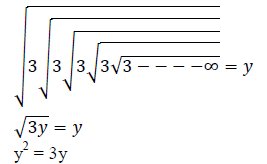y(y-3) = 0
y = 3
Practice Test: Civil Engineering (CE)- 10 - Question 11
In laboratory compaction tests, the optimum moisture content of soil decreases:
Detailed Solution for Practice Test: Civil Engineering (CE)- 10 - Question 11
Optimum moisture content of soil decreases:
(1) With increase in compaction energy
(2) With increase in coarse grains in the soil
Practice Test: Civil Engineering (CE)- 10 - Question 12
For a matrix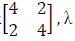=6. The eigen vectors for the matrix are:
Detailed Solution for Practice Test: Civil Engineering (CE)- 10 - Question 12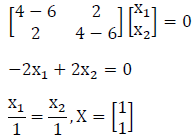Practice Test: Civil Engineering (CE)- 10 - Question 13
The equation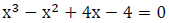is to be solved using the Newton Raphson Method. If x = 2is taken as initial approximation of the solution, then the next approximation using this method is:
Detailed Solution for Practice Test: Civil Engineering (CE)- 10 - Question 13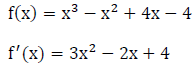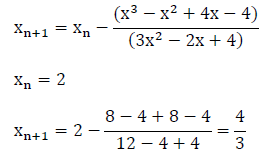Practice Test: Civil Engineering (CE)- 10 - Question 14
A bag contains 5 white and 8 black balls and a second bag contains 3 white and 7 black balls. A ball is drawn at random from the first bag and placed in the second bag. Find the probability that drawn ball is white.
Detailed Solution for Practice Test: Civil Engineering (CE)- 10 - Question 14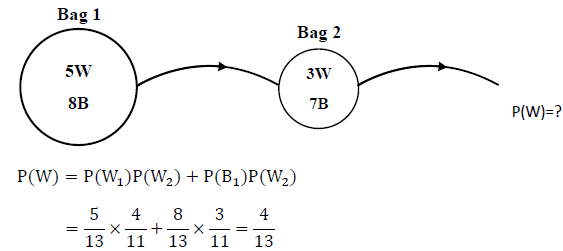Practice Test: Civil Engineering (CE)- 10 - Question 15

The non destructive test on fresh concrete is done using:

Detailed Solution for Practice Test: Civil Engineering (CE)- 10 - Question 15

Pull out Test
The test measures the force required to pull out a previously cast in steel insert with an embedded enlarged end in the concrete. Thus, it is a type of destructible test.
Ultrasonic Test
An ultrasonic pulse velocity (UPV) test is an in-situ, nondestructive test to check the quality of concrete. In this test, the strength and quality of concrete is assessed by measuring the velocity of an ultrasonic pulse passing through a concrete structure.
Radioactive methods of testing concrete can be used to detect the location of reinforcement, measure density and perhaps establish whether honeycombing has occurred in structural concrete units. It is a non-destructible test and is used for hardened concrete rather for fresh concrete.

Practice Test: Civil Engineering (CE)- 10 - Question 16
Curing of concrete by stream under pressure:
1) Increases the compressive strength of concrete
2) Reduces the shear strength of concrete
3) Increases the speed of chemical reaction
Select the correct answer using the codes given below
Practice Test: Civil Engineering (CE)- 10 - Question 17
The presence of entrained air in concrete results in:
i) Reduced bleeding
ii) Lowering density of concrete
iii) Decrease in strength at all ages
Practice Test: Civil Engineering (CE)- 10 - Question 18
According to Whitney’s theory of ultimate load method of concrete, the ultimate strain of concrete is assumed to be:
Practice Test: Civil Engineering (CE)- 10 - Question 19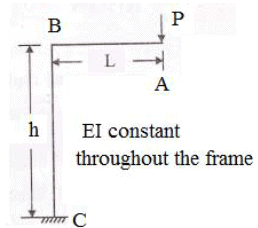A rigid cantilever frame ABC is fixed at C, It carries a point load P at A. Neglecting the axial deformation, the horizontal deflection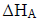of the point A will be:
Detailed Solution for Practice Test: Civil Engineering (CE)- 10 - Question 19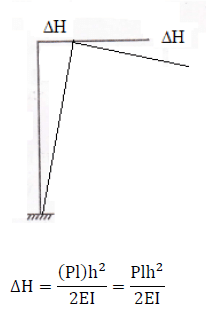Practice Test: Civil Engineering (CE)- 10 - Question 20
For a circular curve of radius 200m, the coefficient of lateral friction is 0.15 and the design speed is 40 kmph. The equilibrium super elevation would be
Detailed Solution for Practice Test: Civil Engineering (CE)- 10 - Question 20
The super elevation at which pressure on outer and inner wheels is same is called equilibrium super elevation.
Friction force = 0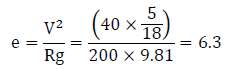Practice Test: Civil Engineering (CE)- 10 - Question 21
The composite sleeper index used for wooden sleepers is the index of:
Practice Test: Civil Engineering (CE)- 10 - Question 22
A ball kept in a closed box moves in the box making collisions with the walls. The box is kept on a smooth surface. The velocity of the centre of mass:
Practice Test: Civil Engineering (CE)- 10 - Question 23
The area on which crops are grown in a particular reason is called:
Detailed Solution for Practice Test: Civil Engineering (CE)- 10 - Question 23
The area on which crops are grown in a particular season is called as seasonal cropped area or seasonal sown area such as Rabi or Kharif area. Net sown area is the total cropped area in the entire year i.e. both the seasons.
Practice Test: Civil Engineering (CE)- 10 - Question 24
If a shaft is subjected to pure torsion, it will fail along the plane:
Detailed Solution for Practice Test: Civil Engineering (CE)- 10 - Question 24
If shaft is subjected to pure torsion it will fail along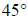to longitudinal axis in brittle material and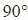to longitudinal axis inductile material.
Practice Test: Civil Engineering (CE)- 10 - Question 25
A 12 hour unit hydrograph of a catchment is triangular in shape with a base width of 64 hours and a peak ordinate of 30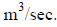If a 2 cm rainfall excess occurs in 12 hour in the catchment, the resulting surface runoff will have:
Detailed Solution for Practice Test: Civil Engineering (CE)- 10 - Question 25
Surface runoff = Area under DRH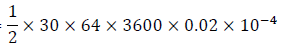6.912 ha m
Practice Test: Civil Engineering (CE)- 10 - Question 26
In Marshall mix design test, the relationship between voids in mineral aggregates and % Bitumen content (BT) is the best illustrated by
Practice Test: Civil Engineering (CE)- 10 - Question 27
Difference between airport and highway pavement thickness arises due to:
2. Tyre pressure
3. Size of vehicle
4. Number of repetition
Which of the statement given above are correct?
Practice Test: Civil Engineering (CE)- 10 - Question 28
One end of a two dimensional water tank has the shape of a quadrant of a circle of radius 2m. When the tank is full the vertical component of the force per unit length on the curved surface will be?
Detailed Solution for Practice Test: Civil Engineering (CE)- 10 - Question 28
Vertical component of force = weight of liquid enclosed above the circular surface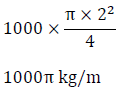Practice Test: Civil Engineering (CE)- 10 - Question 29

A lysimeter is used to measure :

Practice Test: Civil Engineering (CE)- 10 - Question 30
When the recirculation ratio in a high rate trickling filter is unity, then what is the value of recirculation factor?
Detailed Solution for Practice Test: Civil Engineering (CE)- 10 - Question 30
Recirculation factor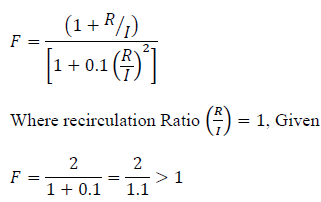Practice Test: Civil Engineering (CE)- 10 - Question 31
Presence of nitrogen in a waste sample is due to the decomposition of:
Detailed Solution for Practice Test: Civil Engineering (CE)- 10 - Question 31
The presence of nitrogen in waste sample is due to the decomposition of proteins
Practice Test: Civil Engineering (CE)- 10 - Question 32
Consider the following activities:
1. Pouring of concrete
2. Erection of formwork
3. Curing of concrete
4. Removal of formwork
What is the correct sequence on a network of these activities?
Detailed Solution for Practice Test: Civil Engineering (CE)- 10 - Question 32
Correct sequence of activities is:
a) Erection of formwork
b) Placing of reinforcement
c) Pouring of concrete
d) Compaction of concrete
e) Removal of formwork
f) Curing
Practice Test: Civil Engineering (CE)- 10 - Question 33
Match List-I (contract types) with List-II (Characteristics):
List-I
a. Labour contract
b.Item rate contract
c. Piecework contract
d.Lump sum contract
List-II
1.Petty works and regular maintenance work
3.Payment made by detailed measurement of different time
4.Not practiced in government
Practice Test: Civil Engineering (CE)- 10 - Question 34
The angle of intersections of the mirrors in an optical square is:
Practice Test: Civil Engineering (CE)- 10 - Question 35
Prying forces can be reduced by:
1) Increasing the end distance
2) Decreasing the end distance
3) Increasing the distance of the bolt from half the root radius for the rolled section Of the above
Practice Test: Civil Engineering (CE)- 10 - Question 36
A bracket carries a load of 120 kN, it is connected to column by means of 2 horizontal fillet welds each of 150 mm effective length and 10mm thickness. The load acts at 80mm from the face of the column as shown in the figure. Find the resultant stress on weld.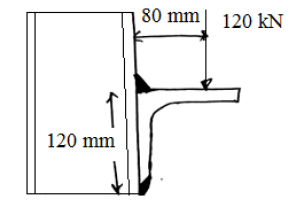Detailed Solution for Practice Test: Civil Engineering (CE)- 10 - Question 36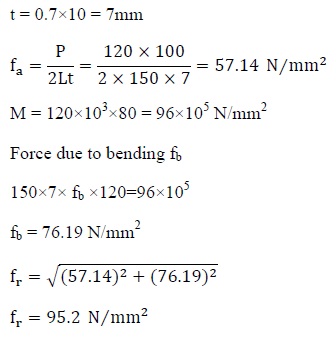Practice Test: Civil Engineering (CE)- 10 - Question 37
A septic tank for a hospital is designed for 250 persons. The flow into the tank may be assumed to be at the rate of 65 lpcd. What would be the surface over loading if following date are given:
Detention period = 20 hours
Depth = 0.9 m
Length: width =1.5
Percolation rate = 15 minutes
Detailed Solution for Practice Test: Civil Engineering (CE)- 10 - Question 37
Quantity of sewage applied = 250×65 = 16250 litres/day
t = 20 hours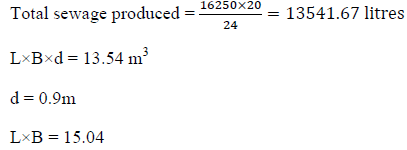B = 3.16 (Since L/B = 1.5)
L = 4.75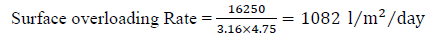Practice Test: Civil Engineering (CE)- 10 - Question 38
A digestion tank of the primary sludge is designed with the help of following data:
I. Average flow = 20MLD
II. Total suspended solids in raw sewage = 300 mg/L
III. Moisture content of the raw sewage =95%
IV. Moisture content of the digested sludge =85%
V. 65% of solids removed in primary tanks
VI. Specific gravity of sludge = 1.02
VII. Digested period = 30 days
Volume of raw sewage produced per day is:
Detailed Solution for Practice Test: Civil Engineering (CE)- 10 - Question 38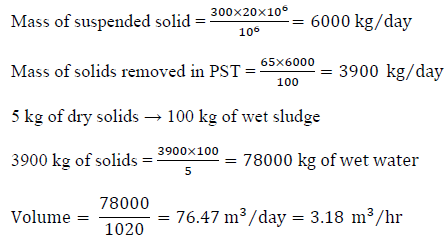Practice Test: Civil Engineering (CE)- 10 - Question 39
What will be the value of limiting depth of neutral axis in a singly reinforced beam of section 300mm × 500mm (effective depth), if strain in concrete at limit state of collapse was found to be 0.0030? Use M20 grade of concrete and Fe 415 grade of steel.
Detailed Solution for Practice Test: Civil Engineering (CE)- 10 - Question 39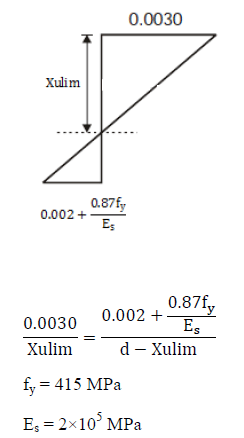d = 500mm
Xulim = 220.70mm
Practice Test: Civil Engineering (CE)- 10 - Question 40
A concrete beam is pre-stressed by a cable carrying an initial pre-stressing force of 300kN. The cross – sectional area of the wires in the cable is 300 mm2. Calculate the ratio of percentage loss of stress in cable only due to shrinkage of concrete due to pre-tensioning and post – tensioning
Assume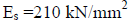Age of concrete at transfer = 8 days
Detailed Solution for Practice Test: Civil Engineering (CE)- 10 - Question 40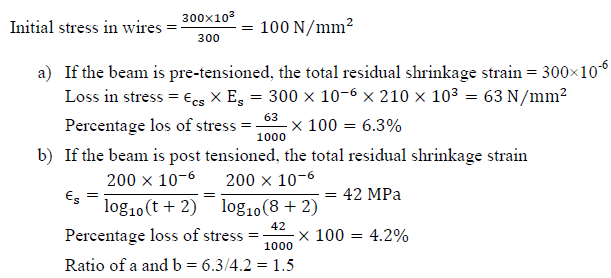Practice Test: Civil Engineering (CE)- 10 - Question 41
Find the critical path for the following data: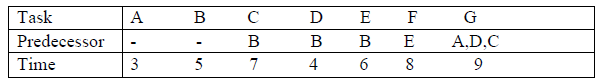Detailed Solution for Practice Test: Civil Engineering (CE)- 10 - Question 41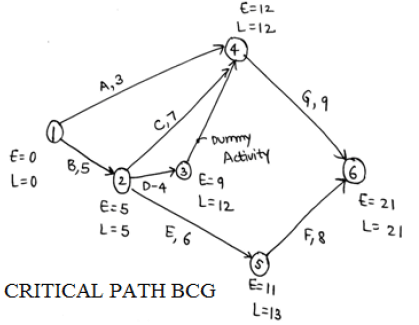Practice Test: Civil Engineering (CE)- 10 - Question 42
Which of the following is not correct?
Practice Test: Civil Engineering (CE)- 10 - Question 43
In the influence line diagram for member AF in the given truss: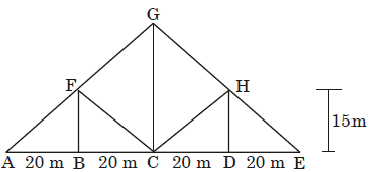Detailed Solution for Practice Test: Civil Engineering (CE)- 10 - Question 43
When unit load is at A
F = 0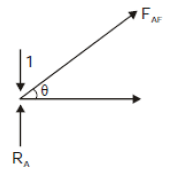When unit load is at B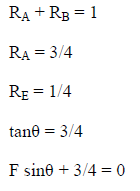F = -1.25
When unit load is at C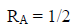F sin? + 1/2 = 0
F = -5/6 = -0.83
Practice Test: Civil Engineering (CE)- 10 - Question 44
Find the magnitude of horizontal displacement at joint E of the truss shown in the figure below due to temperature increase of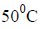in member AC and CE is ________ mm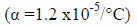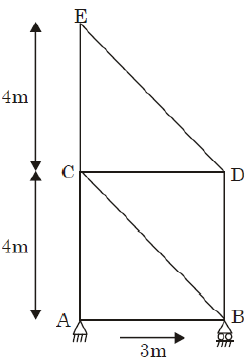Detailed Solution for Practice Test: Civil Engineering (CE)- 10 - Question 44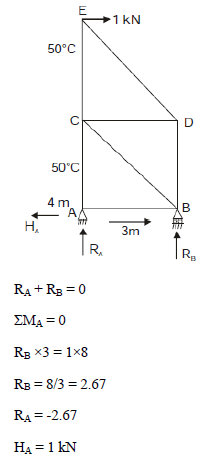Forces in the members are computed: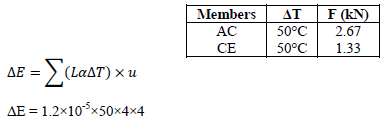= 0.0096 m
= 9.6mm
Practice Test: Civil Engineering (CE)- 10 - Question 45
For the frame shown below: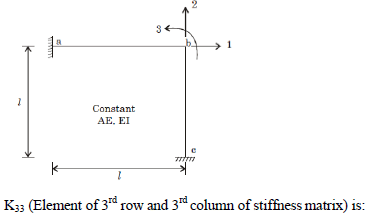Detailed Solution for Practice Test: Civil Engineering (CE)- 10 - Question 45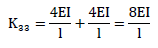Practice Test: Civil Engineering (CE)- 10 - Question 46
A taxiway is to be designed for a supersonic aircraft with a wheel base of 30 m and tread of main loading gear as 6m for a design turning speed of 50 kmph. Assume coefficient of friction between tyre and pavement surface as 0.13 and width of taxiway pavement as 22.5m. Assume oleo distance as 6m. The turning radius of taxiway is:
Detailed Solution for Practice Test: Civil Engineering (CE)- 10 - Question 46
Radius of taxiway will be maximum if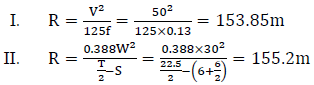III. For supersonic aircraft radius of taxiway should not be less than 180m.
Therefore radius of taxiway will be 180m
Practice Test: Civil Engineering (CE)- 10 - Question 47
An irrigation canal proposed to be excavated in an incoherent alluvial soil of minimum size 0.4mm, for carrying a discharge of 40 cumecs, should have a bed slope of:
Detailed Solution for Practice Test: Civil Engineering (CE)- 10 - Question 47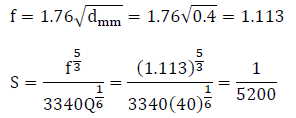Practice Test: Civil Engineering (CE)- 10 - Question 48
A block P of mass 200 g is placed on a block Q of mass 300 g as shown in the figure. Block P is tied to the wall by a massless and inextensible string AB. If the coefficient of static friction for all the surfaces is 0.5, find the minimum force F (in kN) needed to move the block Q is: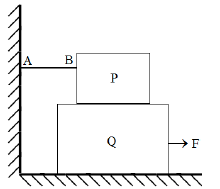Detailed Solution for Practice Test: Civil Engineering (CE)- 10 - Question 48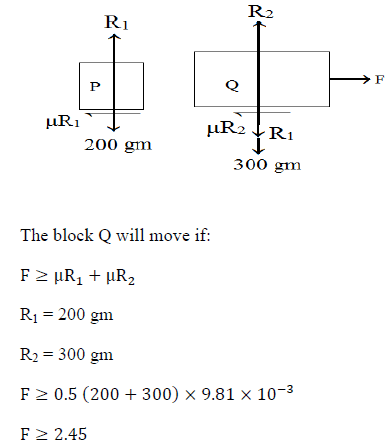Practice Test: Civil Engineering (CE)- 10 - Question 49
A light spring of spring constant K is kept compressed between two blocks of masses m and M on a smooth horizontal surface. When released, the blocks acquire velocities in opposite directions. The spring loses contact with the blocks when it acquires natural length. If the spring was initially compressed through a distance x, find the final speeds of the block having mass M?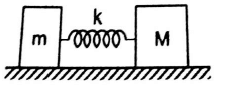Detailed Solution for Practice Test: Civil Engineering (CE)- 10 - Question 49
No external force acts on the system hence linear momentum is constant
Initial momentum is zero as blocks are released from rest
MV - mv = 0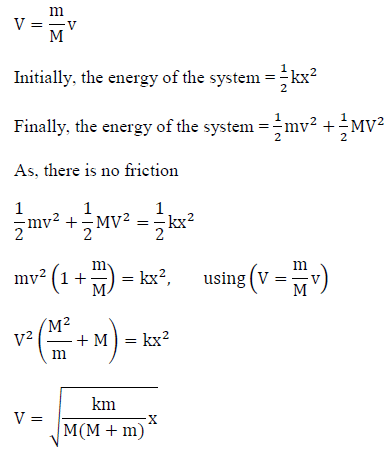Practice Test: Civil Engineering (CE)- 10 - Question 50

Delta (Δ) in cm. Duty (D) in hectare Cumec and Base period (B) in days are related as

Practice Test: Civil Engineering (CE)- 10 - Question 51
Extra widening required at a horizontal curve on a single lane road of radius 100m for a design speed of 60 kmph and for a vehicle with wheel base 6m is:
Detailed Solution for Practice Test: Civil Engineering (CE)- 10 - Question 51
For a single lane road we provide only mechanical widening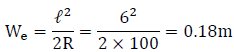Practice Test: Civil Engineering (CE)- 10 - Question 52
The following metrological data pertains to a large reservoir with a watershed area of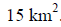The data represents the average values for the day.
Water temperature =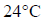Air temperature     =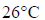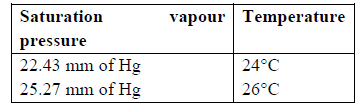Relative humidity = 46%
Find the actual vapour pressure (in mm of Hg)
Detailed Solution for Practice Test: Civil Engineering (CE)- 10 - Question 52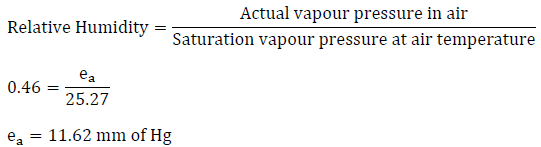Practice Test: Civil Engineering (CE)- 10 - Question 53
Find the directional derivative of the function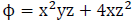at a point (1, -2, -1) in the direction ofDetailed Solution for Practice Test: Civil Engineering (CE)- 10 - Question 53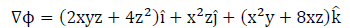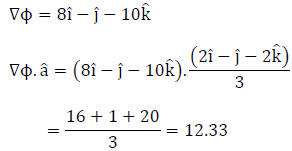Practice Test: Civil Engineering (CE)- 10 - Question 54
Find the value of: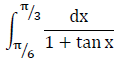Detailed Solution for Practice Test: Civil Engineering (CE)- 10 - Question 54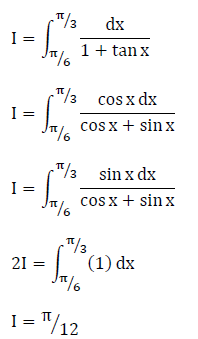Practice Test: Civil Engineering (CE)- 10 - Question 55
A bag contains 5 white, 6 red and 7 black balls, 4 balls are drawn at random. Find the probability that at least 3 balls are black balls.
Detailed Solution for Practice Test: Civil Engineering (CE)- 10 - Question 55
Atleast 3 are black balls = 3 black balls + 4 black balls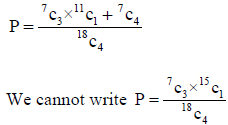Practice Test: Civil Engineering (CE)- 10 - Question 56
A hollow shaft having outer diameter equal to 3 times the inner diameter. It transmits 40 kW power at 2500 RPM. If the maximum permissible shear stress is 32 MPa, then outer diameter is
Detailed Solution for Practice Test: Civil Engineering (CE)- 10 - Question 56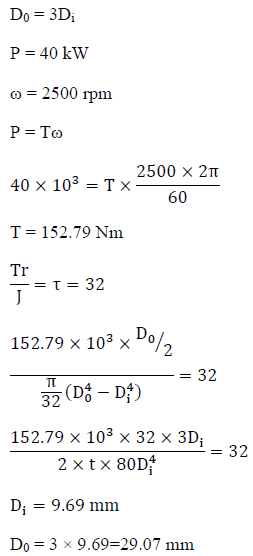Practice Test: Civil Engineering (CE)- 10 - Question 57

The microbial quality of treated piped water supplies is monitored by

Practice Test: Civil Engineering (CE)- 10 - Question 58
A rectangular channel carries a flow of 3.5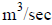the depth of flow being 1.5m and width of channel is 2m. Maximum rise in floor at a section so that upstream flow condition does not change is given as?
Detailed Solution for Practice Test: Civil Engineering (CE)- 10 - Question 58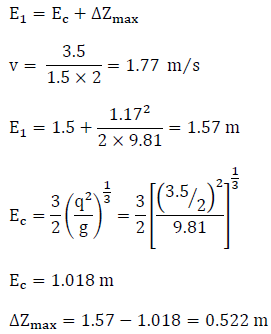Practice Test: Civil Engineering (CE)- 10 - Question 59
The stream function for a 2-D flow is given by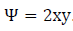.The resultant velocity at a point P(2, 3) is:
Detailed Solution for Practice Test: Civil Engineering (CE)- 10 - Question 59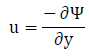=-2x=-4 units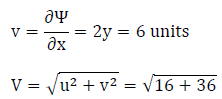= 7.21 units/sec
Practice Test: Civil Engineering (CE)- 10 - Question 60
In a laminar boundary layer, the velocity distribution can be assumed to be given, in usual notation as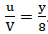Which of the following is the correct expression for the displacement thickness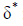for this boundary layer?
Detailed Solution for Practice Test: Civil Engineering (CE)- 10 - Question 60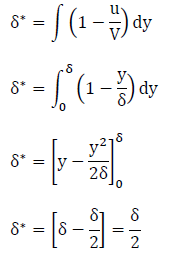Practice Test: Civil Engineering (CE)- 10 - Question 61
A vertical photograph was taken from a height of 3500 m above mean sea level with a camera of focal length 120 mm. IT contains 2 points a and b corresponding to ground points A and B.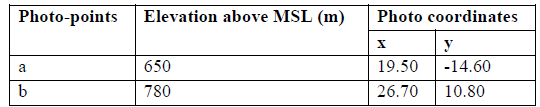For out horizontal length AB
Detailed Solution for Practice Test: Civil Engineering (CE)- 10 - Question 61
Scale for point A: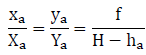Scale for point B: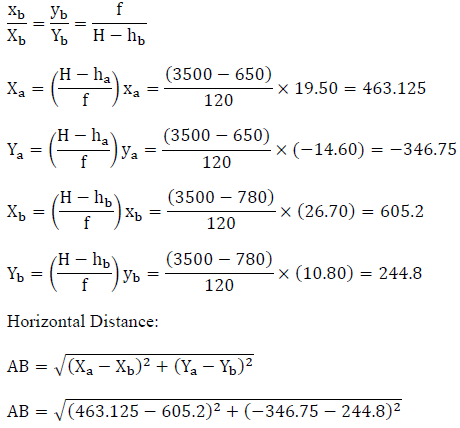AB=608.37 m
Practice Test: Civil Engineering (CE)- 10 - Question 62
For a reciprocal leveling, following readings were taken: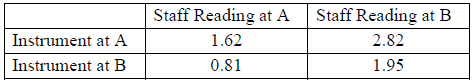If R.L. of point A is 200m and the distance between A and B is 300m. Calculate the angular error in instrument. Neglect effect of curvature and refraction.
Detailed Solution for Practice Test: Civil Engineering (CE)- 10 - Question 62
(2.82 - e) – 1.62 = 1.95 – (0.81 - e)
e = 0.03
Angular error in inclination =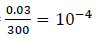Practice Test: Civil Engineering (CE)- 10 - Question 63
In an insitu vane shear test on saturated clay, a torque of 35 Nm was required to shear the soil. The diameter of the vane was 50mm and length 100mm.When the soil was remoulded, the torque required to shear the soil in the remoulded state was 5 Nm. Determine the sensitivity of the clay
Detailed Solution for Practice Test: Civil Engineering (CE)- 10 - Question 63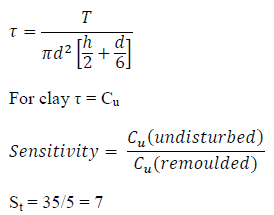Practice Test: Civil Engineering (CE)- 10 - Question 64
A footing 4m × 2mm in plan, transmits a pressure of 150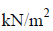on a cohesive soil having E=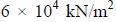and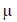==0.5. Determine the immediate settlement of the footing at the center assuming the footing to be rigid footing. Influence factor for flexible footing is 1.52
Detailed Solution for Practice Test: Civil Engineering (CE)- 10 - Question 64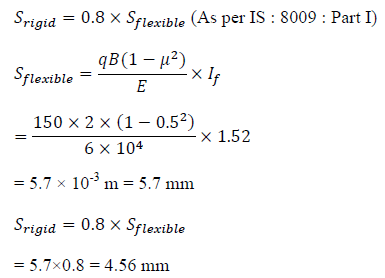Practice Test: Civil Engineering (CE)- 10 - Question 65
A clay sample originally 25mm thick and at a void ratio of 1.120, was subjected to a compressive load. After the clay sample was completely consolidated, its thickness was measured to be 24 mm .Compute the final void ratio.
Detailed Solution for Practice Test: Civil Engineering (CE)- 10 - Question 65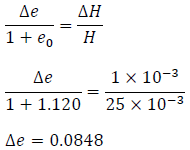Final void ratio = 1.120-0.0848 = 1.0352

## GATE Civil Engineering (CE) 2023 Mock Test Series

27 docs|296 tests
Information about Practice Test: Civil Engineering (CE)- 10 Page
In this test you can find the Exam questions for Practice Test: Civil Engineering (CE)- 10 solved & explained in the simplest way possible. Besides giving Questions and answers for Practice Test: Civil Engineering (CE)- 10, EduRev gives you an ample number of Online tests for practice

## GATE Civil Engineering (CE) 2023 Mock Test Series

27 docs|296 tests(Scan QR code)